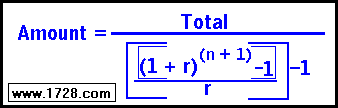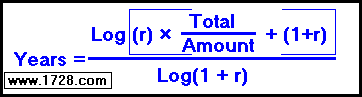***************************

Calculating an Annuity

Before we use the annuity formula, let's solve a short 3 year example the "long way".
First we need the compound interest formula which is:
Total = Principal   ×   ( 1 + Rate )years

Now let's say the amount that we invest annually is \$2,000 per year and the interest rate is 8%.

 The \$2,000 invested 3 years ago has become                   \$2,000 * (1.08)3 = \$2,000 * 1.259712 = \$2,519.424 The \$2,000 invested 2 years ago has become \$2,000 * (1.08)2 = \$2,000 * 1.1664 = \$2,332.80 The \$2,000 invested 1 year ago becomes \$2,000 * (1.08)1 = \$2,000 * 1.08 = \$2,160.00 Adding up all 3 yearly amounts, we obtain \$7,012.22
 As you can see, the mathematics of this can be a little cumbersome especially when the time involved gets larger. To make these calculations a little easier, there is a formula:
 1) Solving the Total Amountwhere the AMOUNT is the annual amount invested each year, 'n' is the number of years and 'r' is the annual rate of the investment. So, we have: \$2,000 * { [(1 + .08)(3 + 1) -1] ÷ .08 } — \$2,000.00 \$2,000 * { [1.36048896 -1] ÷ .08 } — \$2,000.00 \$2,000 * { .36048896 ÷ .08 } — \$2,000.00 \$2,000 * { 4.506112 } — \$2,000.00 \$9,012.224 — \$2,000.00 \$7,012.22 Which is the answer we obtained using the "long" method at the top of this page.

 2) Solving the Annual AmountYou have an 8% annual interest annuity which yields \$100,000, after 25 years. How much has to be invested each year? We input the amounts into the numerator: ([(1.08)(25 + 1)-1] ÷ .08) -1 which equals 78.954415149 Dividing 100,000 by the numerator 100,000 ÷ 78.954415149 = 1266.553615923 which rounds to \$1,266.55 per year.
 3) Solving for YearsYou plan for your retirement by setting up an 8.5% annuity with an annual investment of \$2,500 and you would like this to yield \$500,000 when you retire. How many years will this take? Inputting these numbers into the numerator of the formula: Log(.085 * (500,000 / 2500) + 1.085) = Log(17.085) = 1.2326149831 The log of the denominator 1.085 = 0.035429738185 The number of years = 1.2326149831 ÷ 0.035429738185 = 34.4877 years

RETURN   TO   ANNUITY   CALCULATOR

RETURN   TO   HOME   PAGE